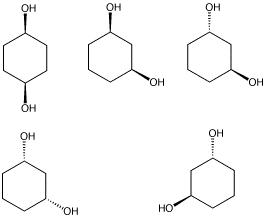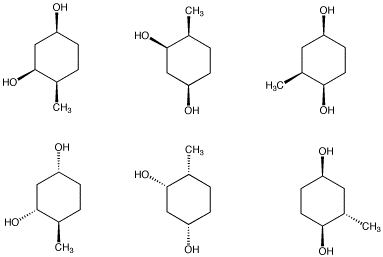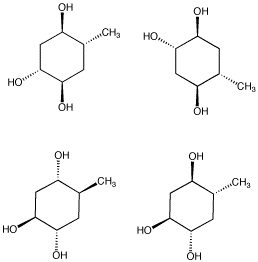SC20. More Practice with Stereochemistry

Optical rotation

Problem SC20.61.

The (+) enantiomer of compound A has an optical rotation of 125o. If a pure sample of compound A has an optical rotation of 100o, what is the composition of the sample?

Problem SC20.62.

The (+) enantiomer of compound B has an optical rotation of 100o. If a pure sample of compound B has an optical rotation of 95o, what is the composition of the sample?

Problem SC20.63.

The (+) enantiomer of compound C has an optical rotation of 18o. If a pure sample of C contains 30% of the (+) enantiomer and 60% of the (-) enantiomer, what is the optical rotation value?

Problem SC20.64.

The (+) enantiomer of compound D has an optical rotation of 25o. If a pure sample of D contains 80% of the (+) enantiomer and 20% of the (-) enantiomer, what is the optical rotation value?

The polarimetry experiment

Problem SC20.61.

A pure sample of the naturally-occurring, chiral compound A (0.050 g) is dissolved in water (2.0 mL) and the solution is placed in a 0.5 dm cell. Three polarimetry readings are recorded with the sample: 0.625o, 0.706o, 0.682o. What is [a]?

Problem SC20.62.

A pure sample of the chiral compound B (0.540 g) is dissolved in ether (2.0 mL) and the solution is placed in a 1.0 dm cell. Three polarimetry readings are recorded with the sample: 1.225o, 1.106o, 1.182o. What is [a]?

Problem SC7.63.

A pure sample of the (+) enantiomer of compound C shows [a] = 42o. What would be the observed a if a solution of the sample was made by dissolving 0.250 g in 2.0 mL of acetontirile and was then placed in a 0.5 dm cell?

Problem SC20.64.

A student has prepared compound D in lab. She is sure the compound contains no impurities; a number of physical analyses have confirmed the structure and purity of the compound. A sample of compound D (0.10 g) is dissolved in methanol (2.0 mL) and the solution is placed in a 1.0 dm cell. Three polarimetry readings are recorded with the sample: 0.995o, 0.904o, 0.936o.

1. What is [a]?
2. The optical rotation of D has previously been reported as 25o. What is the optical purity of this sample?
3. What is the enantiomeric excess of this sample?
4. What is the composition of this sample?
5. Why did the previous analyses show that there was only one compound present?

Problem SC20.65.

Suppose a student prepared a sample of a compound with known [a] = 40. The student did not realize that her sample contained 10% (by mass) of a non-chiral impurity. After correcting for c and l, what did she measure for [a]?

Diastereomers and Physical properties

Problem SC20.101.

From the following group of molecules, select:

1. a pair that are the same compound.
2. a pair that would have the same physical properties but opposite optical activities.
3. a pair that have different physical properties but the same connectivity.
4. a pair that have different connectivity.
5. a compound that contains chiral centers but has no optical activity.Problem SC20.102.

From the following group of molecules, select:

1. a pair that are the same compound.
2. a pair that would have the same physical properties but opposite optical activities.
3. a pair that have different physical properties but the same connectivity.
4. a pair that have different connectivity.Problem SC20.103.

From the following group of molecules, select:

1. a pair that are the same compound.
2. a pair that would have the same physical properties but opposite optical activities.
3. a pair that have different physical properties but the same connectivity.